# Predicting Singapore Pools 4d Lottery winning numbers - Part 2

## Introduction

A little backstory.

I wrote an article on using the TensorFlow library to predict 4D not too long ago. After pusblishing the article, I wanted to further my knowledge on AI literacy but wasn't sure how to.

By chance, I stumbled upon AI Singapore's AI for Industry programme while trying to automate my pizza ordering process.

I decided to enroll given that the programme was part time and online. Furthermore, I qualified for CITREP+ funding.

## The Bet - Part 2

Fast forward, nine months later, Ah Seng approached me when he heard that I completed the programme.

"Oei Terence, tia gong you know a bit of AI liao leh! I lost my job because of Covid leh bro. I'm sure you can predict 4D liao correct? Help leh bro!"

My stance on 4D prediction remains the same. Lottery numbers are random events hence it is a futile process to predict the results.

Ah Seng is a close friend of mine. Since I can't convince him with my words, perhaps the data can.

## Framing The Problem

We are testing a few hypothesis namely:

1. Are we able to predict 4D results?
2. Are we able to determine which prize category a future number will be?
3. Can we predict a number based on a given date?

This may seem like a regression problem initially but is in fact a classification task. We are trying to predict various labels; in this case 0000 - 9999, based on the input data.

## Getting The Data

It's been more than nine months since the previous article. Our 4D historical data is probably outdated. Time to fire up the scraping script! (I'm sorry Singapore Pools LOL!).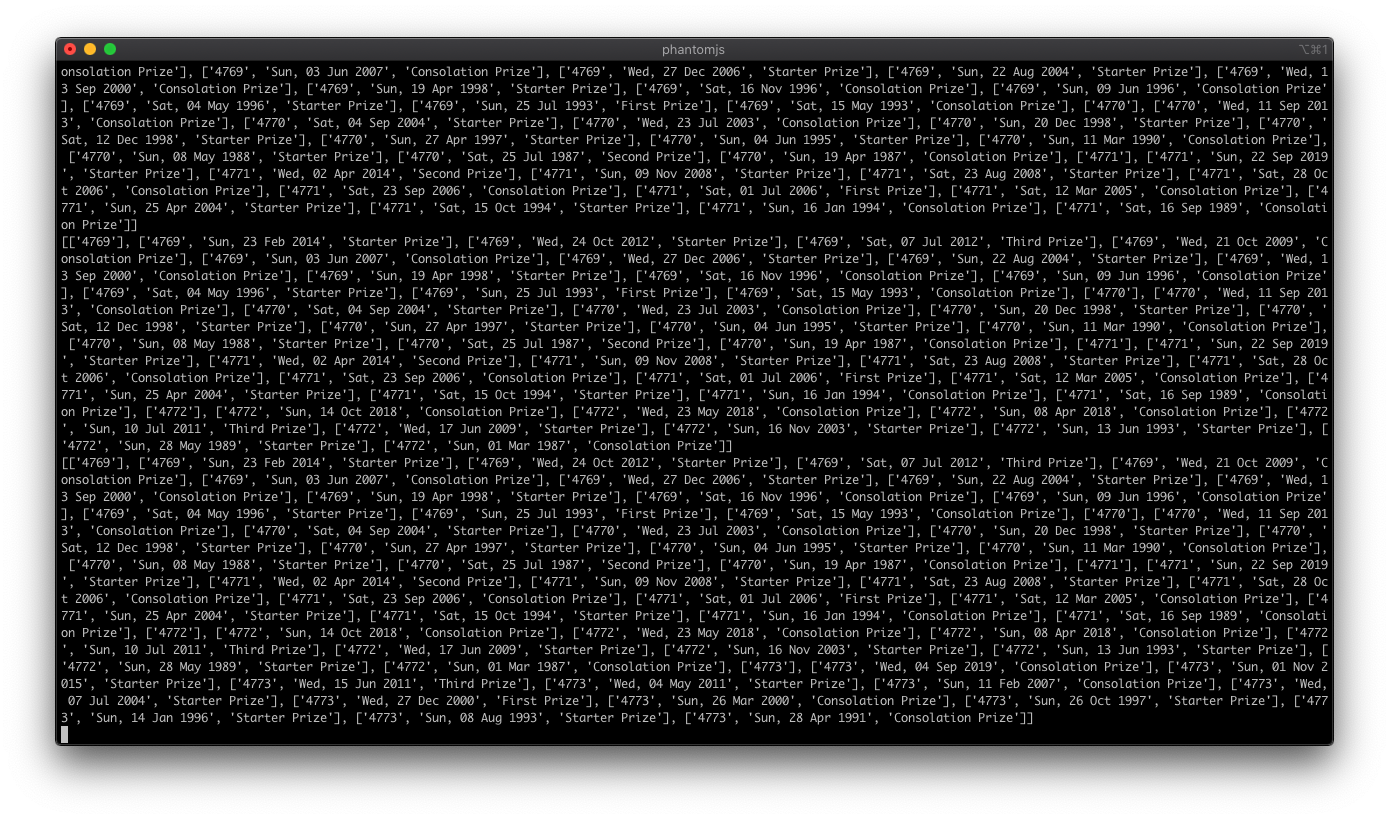Here we go again!

## Gaining Insights From The Data

Let's see if we can gain futher insights from the data. We will be using the Pandas library to perform the EDA (Exploratory Data Analysis).

``````import pandas as pd

>>> len(data)
106946

>>> data.info()
##   Column       Non-Null Count   Dtype
---  ------      --------------   -----
0   number      106946 non-null  int64
1   result      106946 non-null  int64
2   day         106946 non-null  int64

>>> data.number.value_counts()
2967    23
4785    23
9395    23
..

......``````

Based on the observations, we noticed that:

1. The first 4D draw was on 31st May 1986.
2. The total number of draws were 4651 but listed as 4562 on Singapore Pools website.
3. A total of 106946 results has been drawn till date.
4. There used to be draws on Thursdays.

*Data accurate as of 27th June 2020.

## A Few Picture Tells A Thousand Words.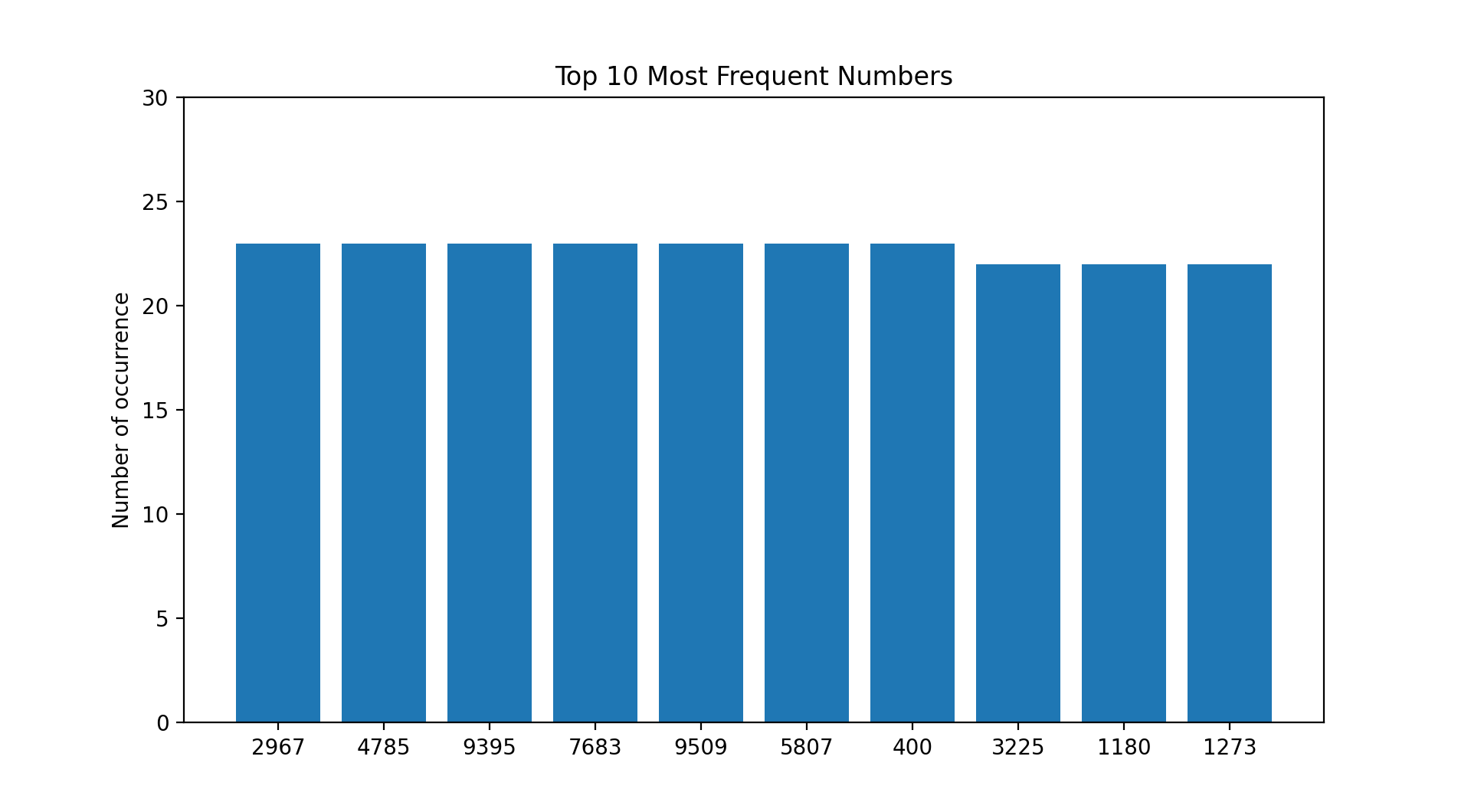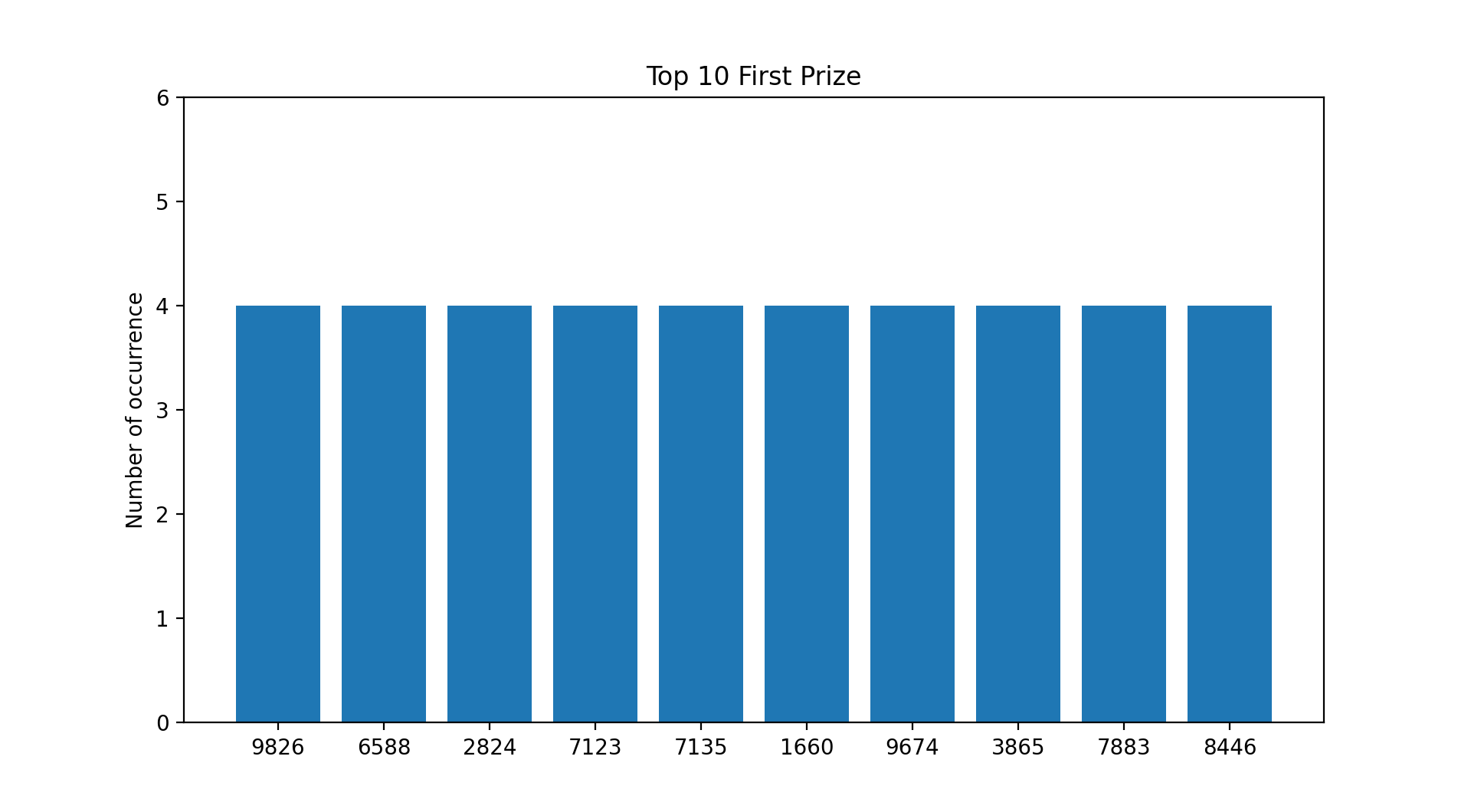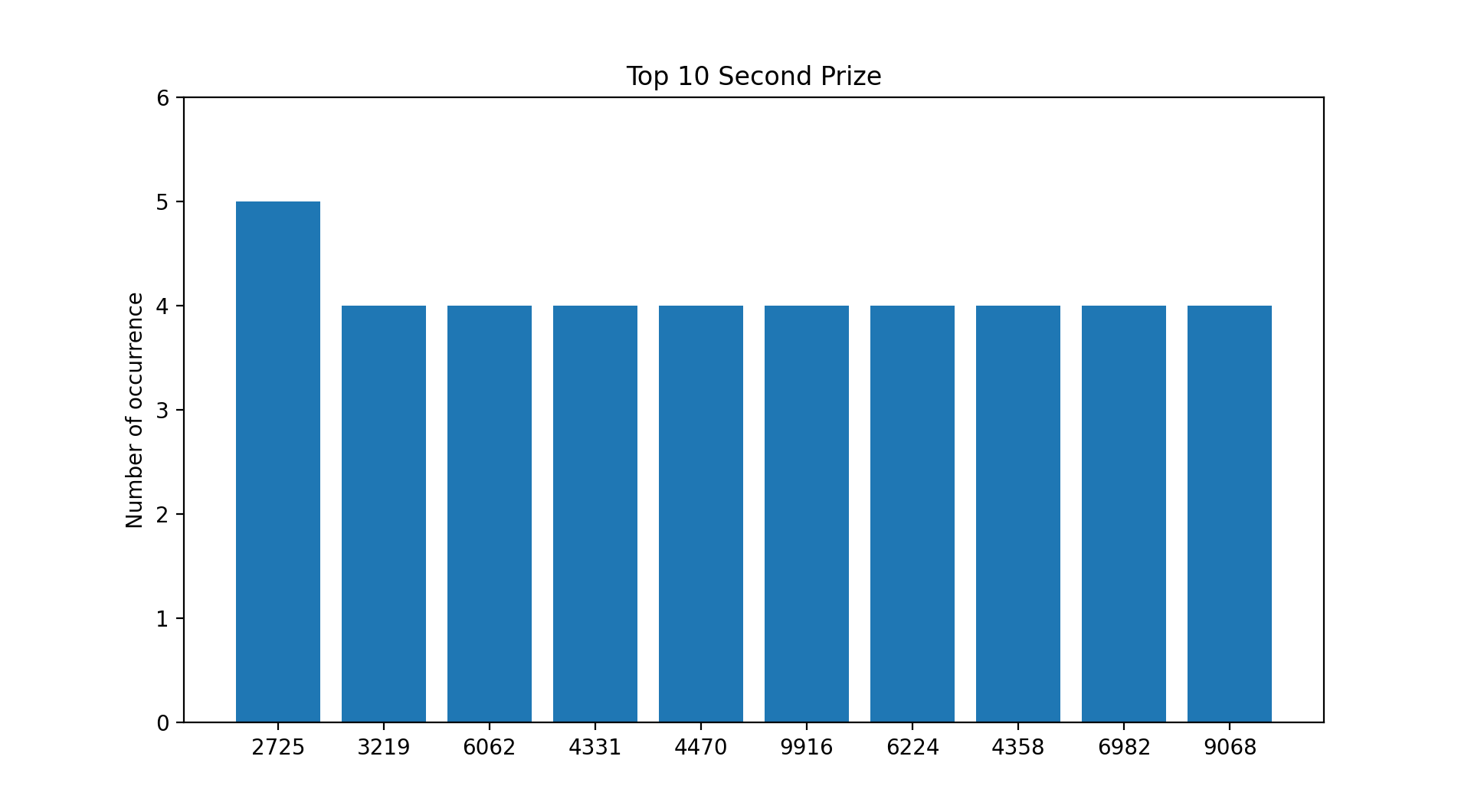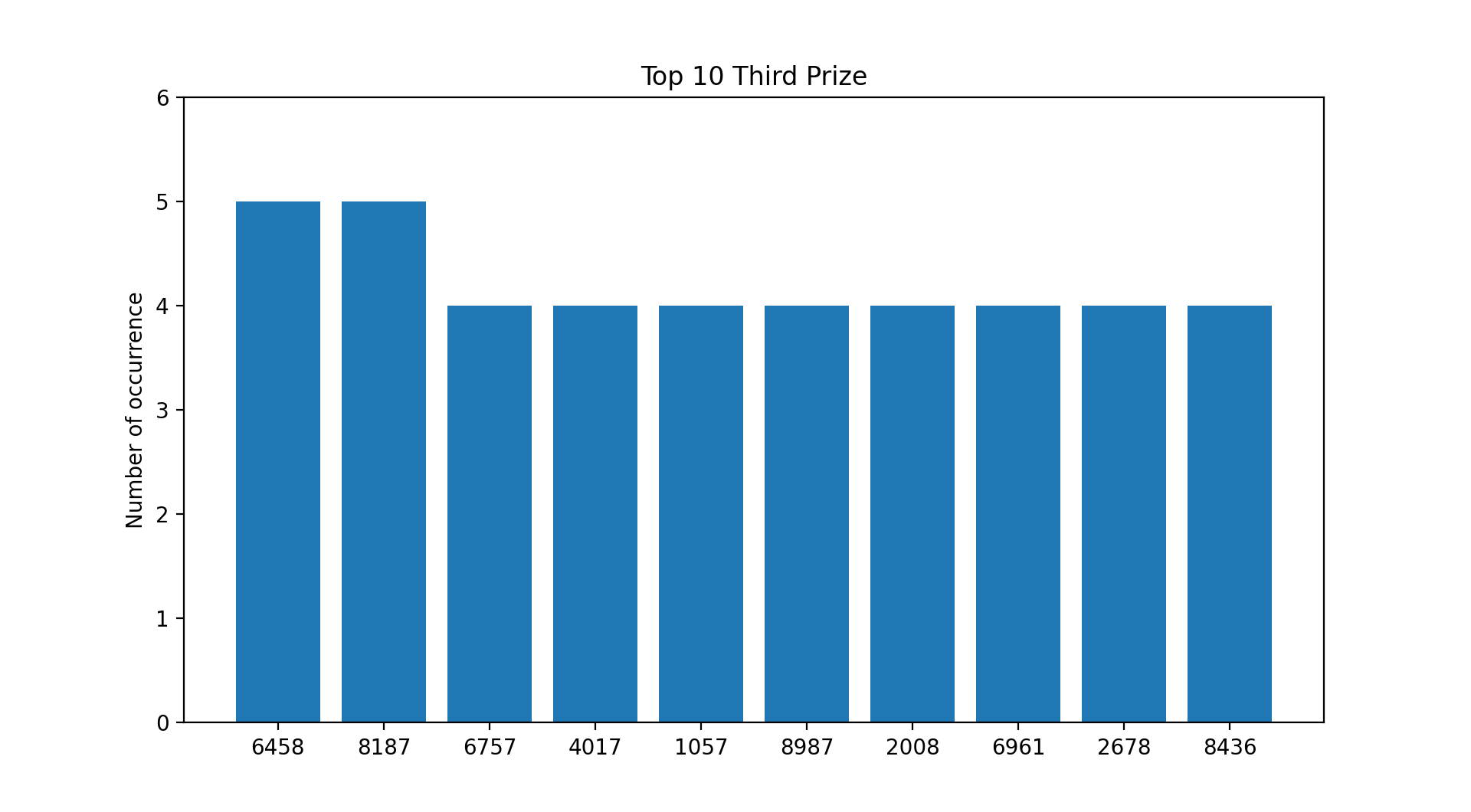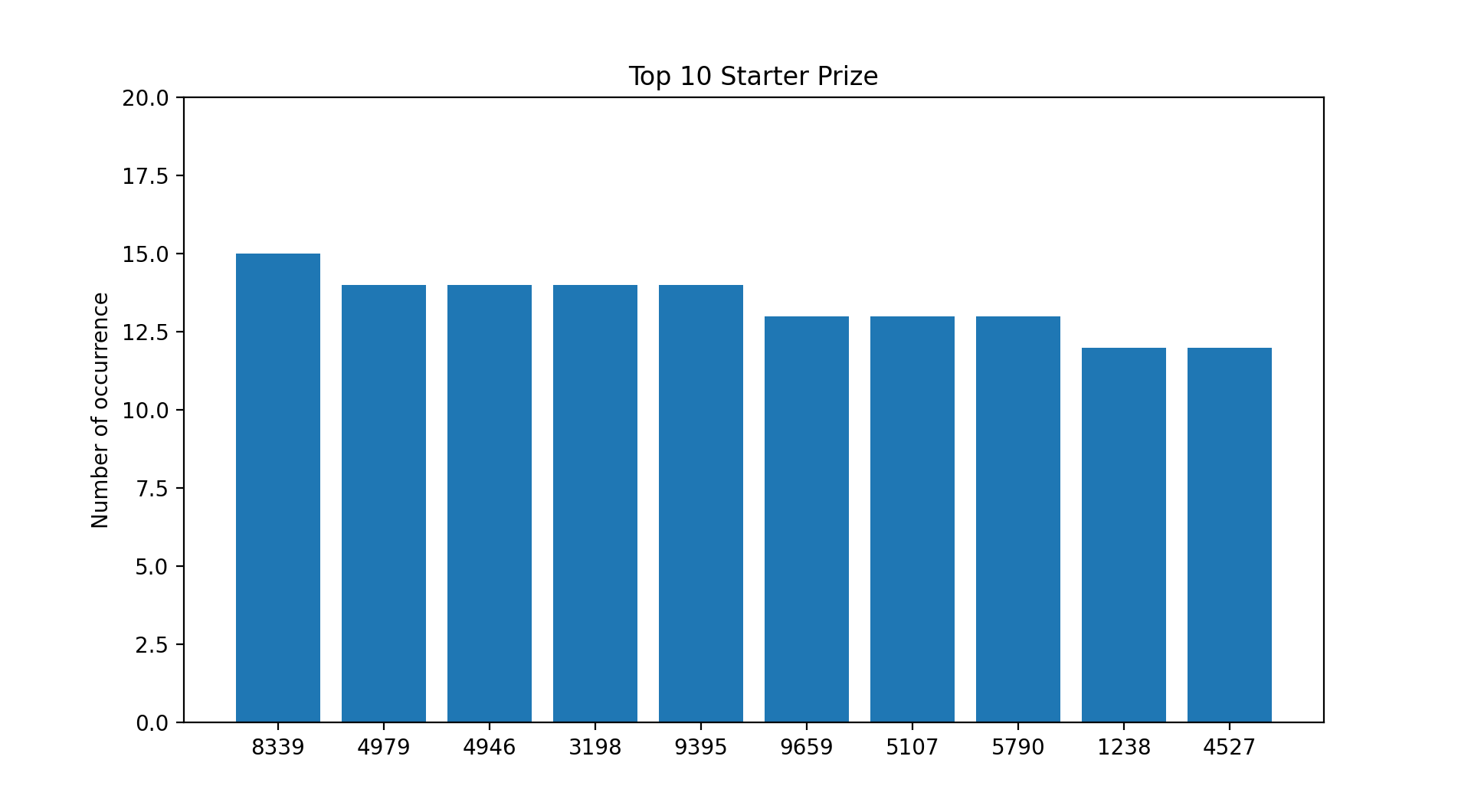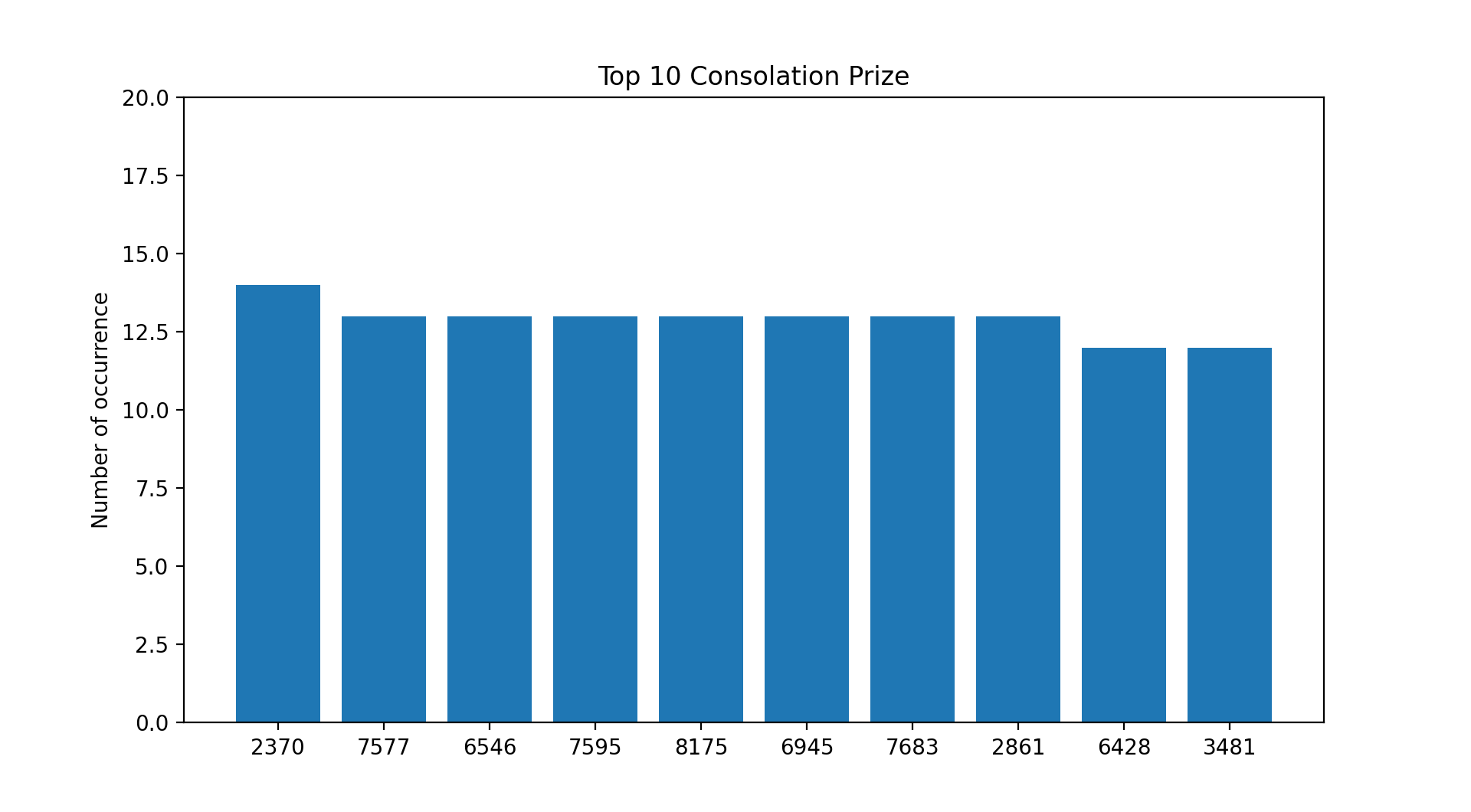Based on the current payouts and the assumption that you placed a \$1 Ordinary Big bet on every draw since 31st May 1986 :

1. You would have recouped your losses if you selected any of the numbers from the first and second prize list.
2. You would have recouped your losses from a few selected numbers from the third prize list.
3. You would have incurred losses if you selected numbers from the consolation prize list.

*Data accurate as of 27th June 2020.

## Pearson’s R

Let's see if there are any correlations among the various attributes.

``````corr_matrix = data.corr()
>>> corr_matrix["number"].sort_values(ascending=False)

number                  1.000000
Sat                     0.007410
Consolation Prize       0.001863
Starter Prize           0.001664
Thu                     0.000563
Third Prize            -0.000045
First Prize            -0.002262
Wed                    -0.002727
Sun                    -0.005029
Second Prize           -0.006265``````

Nope!

## Learning From Sci-Kit Learn

In the original article, I was being ambitious by trying to predict all four numbers based on the input data.

This time around, we split the numbers into four and try to predict each permutation instead.

Let's also perform one-hot encoding on the date and category values.

``````## one-hot encode
data = pd.get_dummies(data, columns=['day_of_week', 'result', 'mm'])

## create permutation columns using string indexing
data['first_perm'] = data['number'].str[0:1]
data['second_perm'] = data['number'].str[1:2]
data['third_perm'] = data['number'].str[2:3]
data['fourth_perm'] = data['number'].str[3:4]

>>> data.info()

##   Column                    Non-Null Count   Dtype
---  ------                    --------------   -----
0   dd                        106946 non-null  int64
1   yyyy                      106946 non-null  int64
2   day_of_week_Sat           106946 non-null  uint8
3   day_of_week_Sun           106946 non-null  uint8
4   day_of_week_Thu           106946 non-null  uint8
5   day_of_week_Wed           106946 non-null  uint8
6   result_Consolation Prize  106946 non-null  uint8
7   result_First Prize        106946 non-null  uint8
8   result_Second Prize       106946 non-null  uint8
9   result_Starter Prize      106946 non-null  uint8
10  result_Third Prize        106946 non-null  uint8
11  mm_Apr                    106946 non-null  uint8
12  mm_Aug                    106946 non-null  uint8
13  mm_Dec                    106946 non-null  uint8
14  mm_Feb                    106946 non-null  uint8
15  mm_Jan                    106946 non-null  uint8
16  mm_Jul                    106946 non-null  uint8
17  mm_Jun                    106946 non-null  uint8
18  mm_Mar                    106946 non-null  uint8
19  mm_May                    106946 non-null  uint8
20  mm_Nov                    106946 non-null  uint8
21  mm_Oct                    106946 non-null  uint8
22  mm_Sep                    106946 non-null  uint8
23  first_perm                106946 non-null  object
24  second_perm               106946 non-null  object
25  third_perm                106946 non-null  object
26  fourth_perm               106946 non-null  object
dtypes: int64(2), object(4), uint8(21)``````

Great! After some munging, we are ready to select and train a Machine Learning model!

We set the 'first_perm' column as the targetted feature. Next, we split our data into a 70/30 train test set and stratify the data based on the 'first_perm' column so as to obtain an even distribution.

``````## Create a dataframe with all training data except the target column
X = df.drop(columns=['first_perm'])

## Separate target values
y = df['first_perm']

## Split data set into 70/30 train test set
X_train, X_test, y_train, y_test = train_test_split(X, y,
test_size=0.3,
random_state=42,
stratify=df['first_perm'])``````

## SGD Classifier

Before proceeding, we normalise the features in the training set using Sci-kit Learn's StandardScaler() function.

This scaler transforms the data and provide equal 'weights' to the distribution. Read Sci-kit Learn StandardScaler.

``````## SGD Classifier
from sklearn.preprocessing import StandardScaler
scaler = StandardScaler()
X_train = scaler.fit_transform(X_train)
X_test = scaler.fit_transform(X_test)
X_train_scaled = scaler.fit_transform(X_train.astype(np.float64))

sgd_clf.fit(X_train_scaled, y_train)
>>> sgd_clf.predict(X_test)

['3' '9' '5' ... '5' '3' '7']``````

Let's evaluate our model using cross-validation.

``````## Cross Validation
from sklearn.model_selection import cross_val_score
y_train_pred = cross_val_score(sgd_clf, X_train_scaled, y_train, cv=5, scoring="accuracy")
>>> y_train_pred

[0.0972417  0.09677419 0.09764894 0.09925194 0.1010553 ]``````

Pathetic! We are getting a 10% accuracy on our predictions.

On closer inspection, it looks about right! We have a 1/10 chance that our model predicts the number correctly (0 - 9).

Perhaps the confusion matrix can help to alleviate the confusion.

``````from sklearn.model_selection import cross_val_predict
y_train_pred = cross_val_predict(sgd_clf, X_train_scaled, y_train, cv=5)

## Focus the plot on the errors instead
from sklearn.metrics import confusion_matrix
conf_mx = confusion_matrix(y_train, y_train_pred)
row_sums = conf_mx.sum(axis=1, keepdims=True)
norm_conf_mx = conf_mx / row_sums

np.fill_diagonal(norm_conf_mx, 0)
plt.matshow(norm_conf_mx, cmap=plt.cm.gray)
plt.show()``````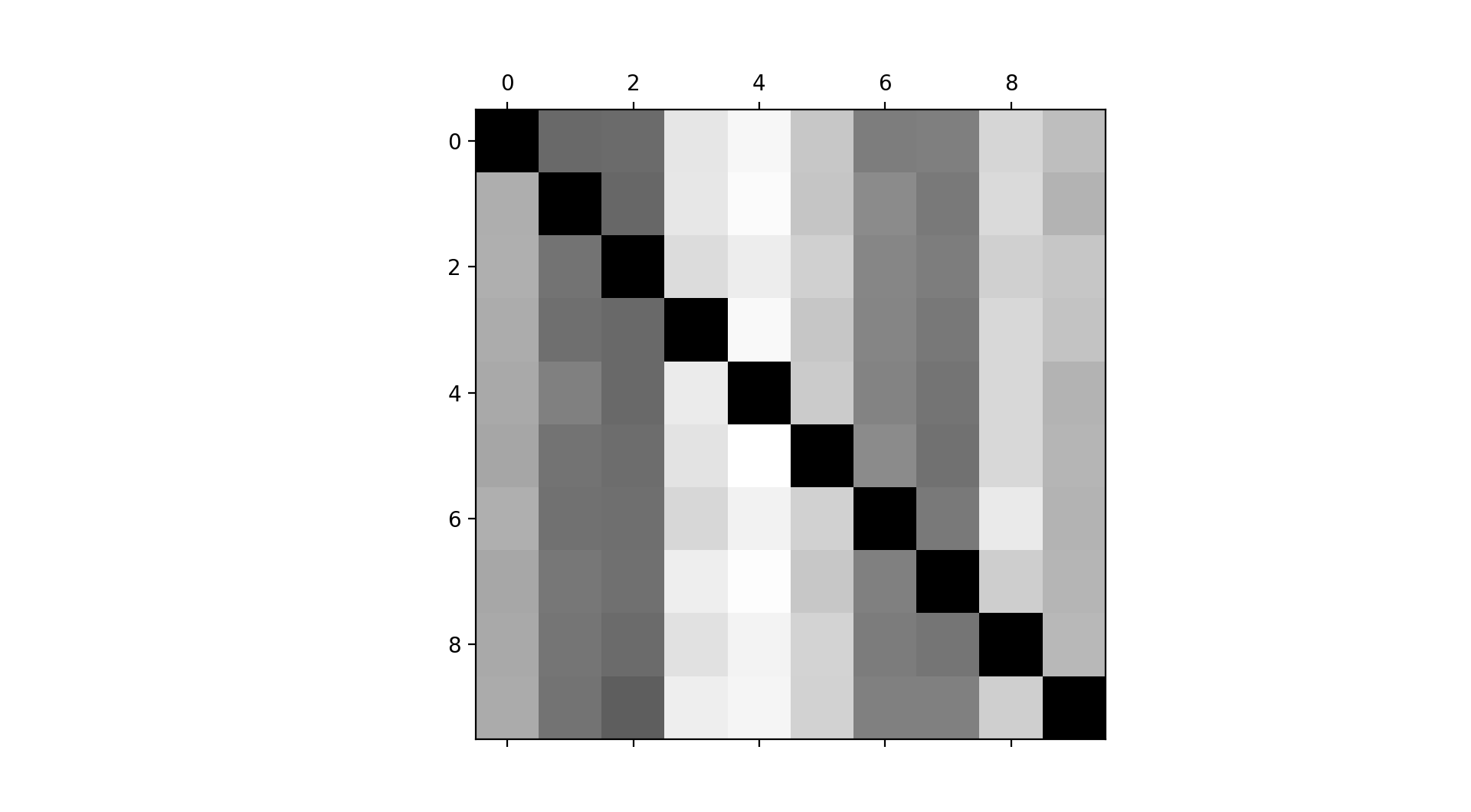From the plot, we see that the predictions were all over the place. There were many misclassification errors, such as the threes, fours, fives and eights as evident from the brighter columns.

## K-Nearest Neighbors

Perhaps our prediction will be better if we used a different classifier.

Let's try the K-Nearest Neighbors classifier or KNN for short.

1. Use the GridSearchCV function to determine the optimal hyperparameter settings.
2. Apply the optimal hyperparameter settings to the classifier.
3. ...
4. Profit!
``````## Fine Tune Hyper Parameters
from sklearn.model_selection import GridSearchCV

k_range = list(range(1,20))
weight_options = ["uniform", "distance"]
param_grid = dict(n_neighbors = k_range, weights = weight_options)
grid = GridSearchCV(knn, param_grid, cv = 10, scoring = 'accuracy')
grid.fit(X,y)

>>> grid.best_score_
0.09973255069648401

>>> grid.best_params_
{'n_neighbors': 7, 'weights': 'uniform'}

## Instantiate KNN classifier with best parameters
knn = KNeighborsClassifier(n_neighbors=7, weights='uniform')
knn.fit(X_train_scaled, y_train)

## Show first 5 model predictions on the test data
k_predict = knn.predict(X_test)[0:5]
>>> k_predict
['0' '7' '2' '1' '7']

## Check accuracy of our model on the test data
knn_score = knn.score(X_test, y_test)
>>> knn_score
0.10207580102231642``````

No surprises there. The accuracy score is about the same as the SGD classifier. I.e. 1 in 10 accuracy.

## Selling Snake Oil

To solidify the idea that 4D results are random, let's use our model to predict the draw held on Sunday, 28th June 2020.

We will test for three scenarios.

1. What will be the first permutation for the first prize?
2. Which prize category will the number 9999 fall under?
3. Will the number 2015 fall under the First Prize category?
``````## SGD Classifier
from sklearn.linear_model import SGDClassifier
sgd_clf = SGDClassifier(random_state=99)
sgd_clf.fit(X_train, y_train)

>>> sgd_clf.predict(number_prediction)
['9']

>>> sgd_clf.predict(consolation_prize_prediction)
['1']

>>> sgd_clf.predict(2015_first_prize_prediction)
['0']``````

The model predicted that:

1. The first number permutation for the first prize result is 9. It's 2.
2. The number 9999 falls under the Consolation Prize category. (Psst, there were no results for 9999 for that particular draw.)
3. The number 2015 does not appear under the First Prize category. Even if it does, this is a flawed representation as any number will fall under one of the prize categories based on the model.## Conclusion

It is the same old story. Can we use AI to predict lottery numbers?

The short answer is No. The long answer is No No No.

We are living in a challenging and stressful period. The Covid 19 pandemic has affected Singaporeans and many had lost their jobs.

Due to these circumstances, many who struggle and are facing financial hardship resort to various means (e.g. buying into 4D prediction scams to name a few) to provide for their families.

The Singapore government launched numerous initiatives to help Singaporeans land a job or boost their career search. Some good resources are My Careers Future and SGUnited jobs.

I know the people working behind the scenes and can vouch for their persistence at helping Singaporeans seek employment.

Bro Ah Seng, if you are reading this, why not give it a shot?

Disclaimer: This is not a paid sponsorship.

“Those who work their land will have abundant food, but those who chase fantasies have no sense.” - Proverbs 12:11